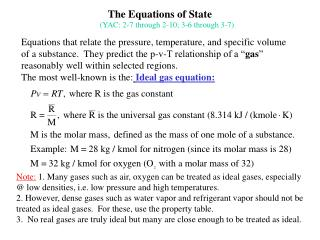DownloadDownload PresentationThe Equations of State

# The Equations of State

Télécharger la présentation## The Equations of State

- - - - - - - - - - - - - - - - - - - - - - - - - - - E N D - - - - - - - - - - - - - - - - - - - - - - - - - - -
##### Presentation Transcript

1. The Equations of State (YAC: 2-7 through 2-10; 3-6 through 3-7) Equations that relate the pressure, temperature, and specific volume of a substance. They predict the p-v-T relationship of a “gas” reasonably well within selected regions. The most well-known is the:Ideal gas equation: Note: 1. Many gases such as air, oxygen can be treated as ideal gases, especially @ low densities, i.e. low pressure and high temperatures. 2. However, dense gases such as water vapor and refrigerant vapor should not be treated as ideal gases. For these, use the property table. 3. No real gases are truly ideal but many are close enough to be treated as ideal.

2. Non-ideal gas behavior T Ideal gas behavior ( high temp) Ideal gas behavior (low pressure) v Ideal Gas Behavior • Most gases: • Deviate from ideal gas in the vicinity of the critical point • and saturated vapor line • Behave as ideal gases at high temperature and low pressure regions • See Figure 2-37, YAC.

3. Compressibility Factor Compressibility factor, Z, is a measure of deviation from the ideal gas. Where Note: Z = 1 for an ideal gas. As Z approaches 1 for a gas at given conditions, the behavior of the gas approaches ideal gas behavior. Although, different gases have very different specific properties at various conditions; all gases behave in a similar manner relative to their critical pressure, Pcr and critical temparature, Tcr. Hence, the gas pressures and temperatures are normalized by the critical values to obtain the reduced pressure, Pr and temperature temparature, Tr. defined as Pr=P/Pcr ; Tr=T/Tcr The reduced values can then be used to determine Z using the Generalized Compressibility Charts (YAC: Fig. 2-40 and A-13)

4. Fig A-13 Generalized Compressibility Charts Example: Steam at T=310° C, P=10 MPa, Tcr=647.3K, Pcr=22.09 MPa (From Table A-1, p. 842); Tr=0.9, Pr=0.45, Z=0.75, not an ideal gas